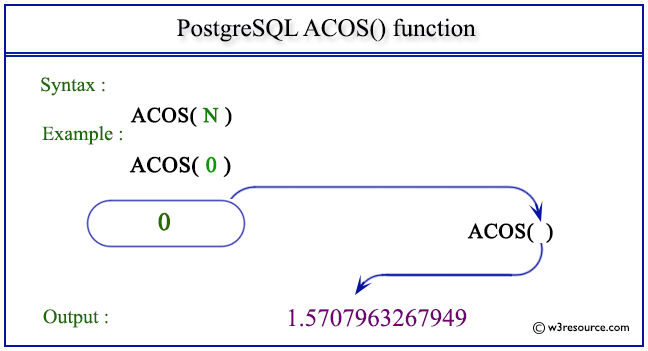# PostgreSQL ACOS() function

## ACOS() function

The PostgreSQL acos() function is used to return the inverse cosine of a given argument.

Syntax:

```acos(a)
```

PostgreSQL Version: 9.3

Pictorial presentation of PostgreSQL ACOS() functionExample 1: PostgreSQL ACOS() function

Code:

``````SELECT ACOS(0) AS "Acos (0)";
```
```

Sample Output:

```    Acos (0)
-----------------
1.5707963267949
(1 row)
```

Example 2: PostgreSQL ACOS() function

Code:

``````SELECT ACOS(1) AS "Acos (1)";
```
```

Sample Output:

``` Acos (1)
----------
0
(1 row)
```

Example 3: PostgreSQL ACOS() function

Code:

``````SELECT ACOS(-1) AS "Acos (-1)";
```
```

Sample Output:

```    Acos (-1)
------------------
3.14159265358979
(1 row)
```

Previous: ABS function
Next: ASIN function

﻿